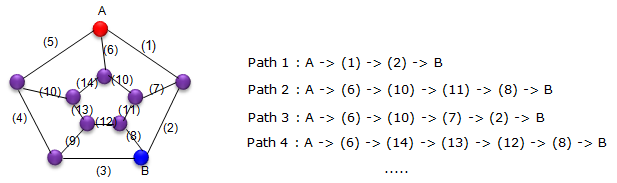Engineering Math - Quick Reference                                 Home : www.sharetechnote.com Graph Theory - Terminology   In this page, I will briefly describe on the list of terminologies that are commonly used in Graph Theory. I will explain directly on this page about the simple concept, but I will write a separate pages that would require long/detailed explanation.       < Vertex and Edge >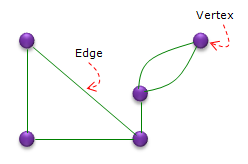< Degree >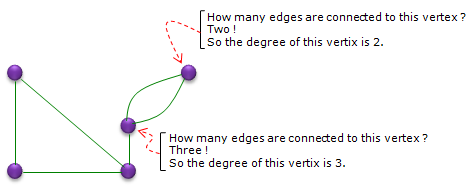< Size >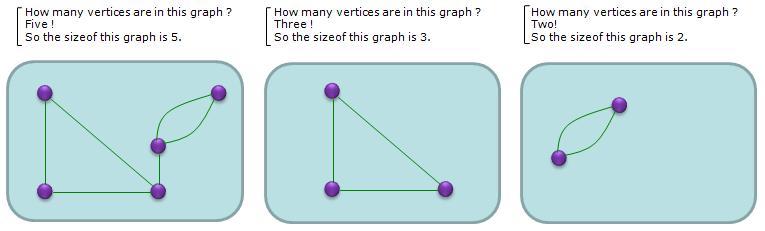< Path and Cycle >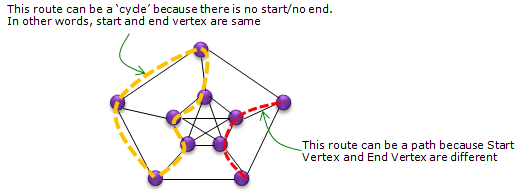< Hamiltonion Path/Cycle >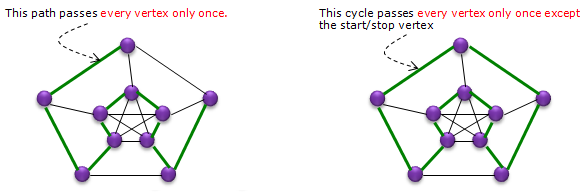< Eulerian Cycle/Circuit >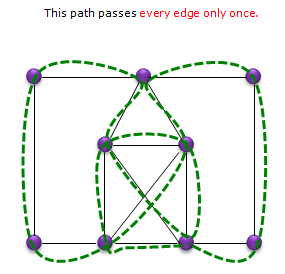< Distance >   Distance is the number of edges connecting two vertices. If there are multiple paths connecting the two vertices, the number of edges on the shortest path (minimum number of edges between the vertices) becomes the distance.   For example, there are many different paths connecting the vertices A and B. But the number of edges on path 1 are used to calculate the distance because it goes through the least number of edges between A and B. The distance between A and B is 2 in this example.